Courses

# Mathematics Test 11 - Straight Line, Circle

## 30 Questions MCQ Test Mock Test Series for JEE Main & Advanced 2022 | Mathematics Test 11 - Straight Line, Circle

Description
This mock test of Mathematics Test 11 - Straight Line, Circle for JEE helps you for every JEE entrance exam. This contains 30 Multiple Choice Questions for JEE Mathematics Test 11 - Straight Line, Circle (mcq) to study with solutions a complete question bank. The solved questions answers in this Mathematics Test 11 - Straight Line, Circle quiz give you a good mix of easy questions and tough questions. JEE students definitely take this Mathematics Test 11 - Straight Line, Circle exercise for a better result in the exam. You can find other Mathematics Test 11 - Straight Line, Circle extra questions, long questions & short questions for JEE on EduRev as well by searching above.
QUESTION: 1

Solution:
QUESTION: 2

### If the point P(x,y) be equidistant from the points A(a b, b–a) and B(a–b,a b), then

Solution:
PA=PB
take square both side
PA^2=PB^2

now use distance
formula ,
{x-(a+b)}^2+{y-(b-a)}^2={x-(a-b)}^2+{y-(a+b)}^2

=>x^2+(a+b)^2-2x(a+b)+y^2+(b-a)^2-2y(b-a)y=x^2+(a-b)^2-2x(a-b)+y^2+(a+b)^2-2y(a+b)

=>2x(a-b)-2x(a+b)=2y(b-a)-2y(a+b)

=>2x{a-b-a-b}=2y{b-a-a-b}

=>2x(-2b)=2y(-2a)

=>bx=ay
QUESTION: 3

### The points (0,8/3),(1,3) and (82,30) are the vertices of

Solution:
QUESTION: 4

P(3,1),Q(6,5) and R(x,y) are three points such that the angle PRQ is a right angle and the area of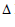RPQ= 7, then the number of such point R is

Solution:
QUESTION: 5

Determine the ratio in which the line 3x + 4y - 9 = 0 divides the line segment joining the points (1,3) and (2,7).

Solution:
QUESTION: 6
Given four lines with equations x+2y–3 = 0, 3x+4y–7 = 0, 2x+3y–4 = 0, 4x+5y–6 = 0, then
Solution:
QUESTION: 7

......PS be the median of the triangle with vertices P(2,2), Q(6,–1) and R(7,3). The equation of the line passing through (1,–1) and parallel to PS is

Solution:
QUESTION: 8
The line 4x+y =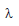cuts the axis of co-ordinates at A and B. If C is the foot of perpendicular drawn from origin O, then AC : CB =
Solution:
QUESTION: 9
Points on the line x+y = 4 that lie at a unit distance from the line 4x+3y–10 = 0 are
Solution:
QUESTION: 10
A line passes through (2,2) and is perpendicular to the line 3x+y = 3. Its y intercept is
Solution:
QUESTION: 11
The four lines ax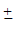byc=0 enclose a
Solution:
QUESTION: 12
The reflection of the point(4,–13) in the line 5x+y+6= 0 is
Solution:
QUESTION: 13
The equation of the bisector of the acute angle between the lines 3x–4y+7 = 0 and 12x+5y–2 = 0 is
Solution:
QUESTION: 14
The vertices of a triangle ABC are (1,1), (4,–2) and (5,5) respectively. Then equation of perpendicular dropped from C to the internal bisector of angle A is
Solution:
QUESTION: 15
A line is such that its segements between the straight lines 5x–y = 4 and 3x+4y–4 = 0 is bisected at the point (1,5). Its equation is
Solution:
QUESTION: 16

The radius of the circle whose centre is on y-axis and which passes through the points (5,2) and (7,–4) is

Solution:
QUESTION: 17
Two circles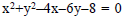and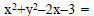0 are such that they
Solution: These are the equation of two circles.The best way to find out whether two circles touches or cuts or do not intersect is to find out the distance between their centres.If this distance is greater than the sum of their radii they don't touch,if equal to sum of radii they just touch, and if less than sum of radii then intersect at two points. If distance is zero then they are concentric.
QUESTION: 18
3x+4y–7 = 0 is common tangent at (1,1) to two equal circles of radius 5. Their centres are the points
Solution:
QUESTION: 19

The points (2,3),(0,2),(4,5)and (0,c) are concyclic if the value of c is

Solution:
QUESTION: 20
Equation of the diameter of the circle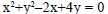which passes through the origin is
Solution:
QUESTION: 21
Which of the following lines is a normal to the circle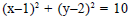Solution:
QUESTION: 22
The equation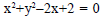represents
Solution:
QUESTION: 23

The coordinates of the point on the circle x2+y2-12x-4y+30=0 which is the farthest from the origin are

Solution:
Centre of the given circle is - (-12/2) , - ( -4/2)
This means (6,2) is the centre of the circle.
The farthest point from the origin on the circle has to be the far end of diameter got by joining (0,0) and (6,2)
Equation of line joining these two points is by two point formula
We have x = 3 y
The point of intersection of this line and circle is got by solving these two
We get points (3,1) and (9,3)
Hence required farthest is (9,3)
QUESTION: 24
The angle between two tangents from the origin to the circle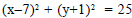is
Solution:
QUESTION: 25
The number of tangents that can be drawn from the point (8,6) to the circle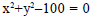is
Solution:
QUESTION: 26
The equation of the common tangent to the circle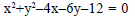,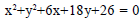at their point of contact is
Solution:
QUESTION: 27
The intercepts made by the circle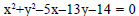on the x-axis and y-axis are respectively
Solution:
QUESTION: 28
The circle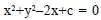touches x-axis, then c =
Solution:
QUESTION: 29
The equation of the passing through the intersection of the circles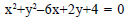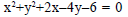and having its centre on the line y = x is
Solution:
QUESTION: 30

The vertex A of a triangle ABC is the point (-2, 3) whereas the line perpendicular to the sides AB and AC are x – y – 4 = 0 and 2x – y – 5 = 0 respectively. The right bisectors of sides meet at P(3/2 , 5/2) . Then the equation of the median of side BC is

Solution: